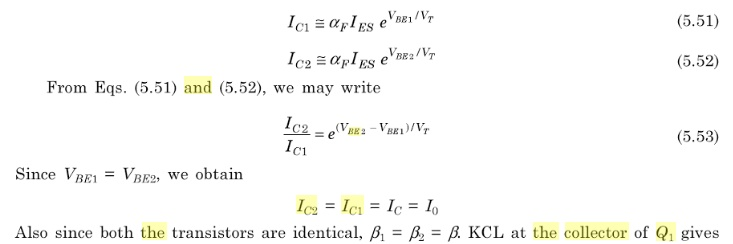Home | | Linear Integrated Circuits | Current Mirror and Current Sources

# Current Mirror and Current Sources

Constant current source(Current Mirror): A constant current source makes use of the fact that for a transistor in the active mode of operation, the collector current is relatively independent of the collector voltage.

CURRENT MIRROR AND CURRENT SOURCES:

Constant current source(Current Mirror):

A constant current source makes use of the fact that for a transistor in the active mode of operation, the collector current is relatively independent of the collector voltage. In the basic circuit shown in fig 1Transistors Q1&Q2 are matched as the circuit is fabricated using IC technology. Base and emitter of Q1&Q2 are tied together and thus have the same VBE. .In addition, transistor Q1 is connected as a diode by shorting it s collector to base. The input current Iref flows through the diode connected transistor Q1 and thus establishes a voltage across Q1.

This voltage in turn appears between the base and emitter of Q2 .Since Q2 is identical to Q1, the emitter current of Q2 will be equal to emitter current of Q1 which is approximately equal to Iref

As long as Q2 is maintained in the active region ,its collector current IC2=Io will be approximately equal to Iref .

Since the output current Io is a reflection or mirror of the reference current Iref, the circuit is often referred to as a current mirror.

Analysis:

The collector current IC1 and IC2 for the transistor Q1 and Q2 can be approximately expressed asFrom equations b>>1, b/(b+2is almost unity and the output current I0 is equal to the reference current, Iref which for a given R1 is constant. Typically Io varies by about 3% for 50 ≤ b ≤200.

It is possible to obtain current transfer ratio other than unity simple by controlling the area of the emitter-base junction (EBJ) of the transistor Q2 . For example, if the area of EBJ of Q2 is 4 times that of Q1,then

IO=4 Iref

The output resistance of the current source is the output resistance,r0 of Q2,

The circuit however operates as a constant current source as long as Q2 remains in the active region.

Study Material, Lecturing Notes, Assignment, Reference, Wiki description explanation, brief detail
Linear Integrated Ciruits : IC Fabrication and Circuit Configuration for Linear ICs : Current Mirror and Current Sources |